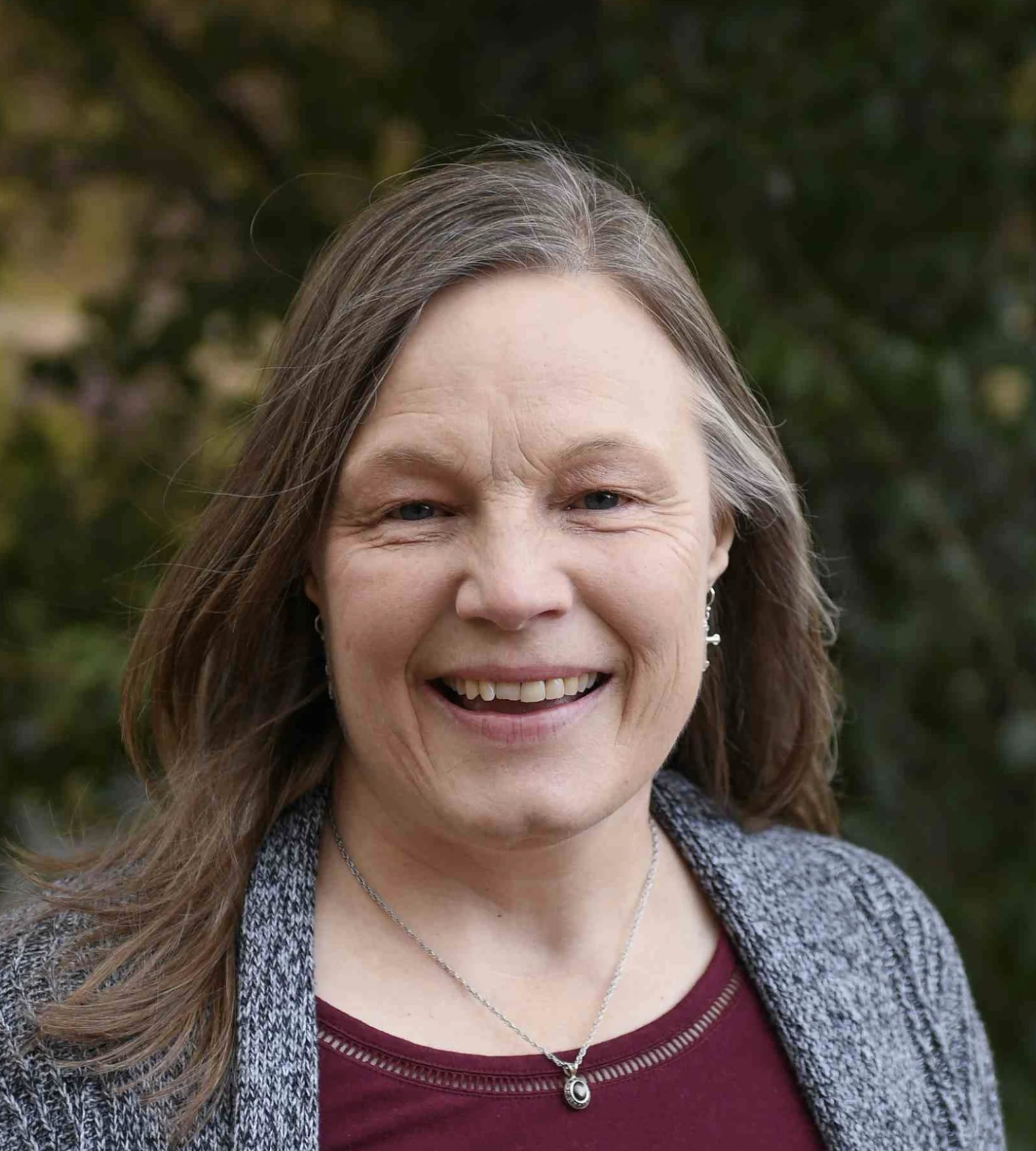# Precalculus with Trigonometry

\$550.00/year
Precalculus with Trigonometry
This class is currently archived, but if you're interested in it being taught again, you can express your interest here!
09/06/2021 - 05/13/2022
Full Year
1.0 credits in Mathematics

Taught by:Lori Miller

In the book Restoring All Things, Warren Cole Smith and John Stonestreet state, “…we glorify [God] by learning of Him and His world. To learn is an act of worship.” In the words of Galileo Galilei, “Mathematics is the language in which God has written the universe.” Learning mathematics is a powerful way to worship God.

Precalculus links together both review and extension of concepts in algebra, geometry, and functions, and serves as a preparation for calculus. Topics covered will include a review of basic algebra; linear, quadratic, and radical equations and inequalities; relations and functions; polynomial and rational functions; exponential and logarithmic functions; trigonometric functions, trigonometric identities; applications of trigonometry; systems of equations and matrices; conic sections; and sequences.

This 32-week course includes a weekly 1.75 hour recitation session. Students will be required to watch CTC Math videos and complete practice questions before class so they are prepared for in-class discussions and activities. Grades will be based on homework, class participation, unit tests, mid-term and final exams, and short oral presentations each semester.

### Course Objectives:

1. Students will recognize that mathematics is a beautiful God-given resource.
2. Students will continue to learn to think mathematically.
3. Students will learn algebraic and trigonometric concepts.
4. Students will apply mathematical concepts to real life situations.
5. Students will discuss the concepts being learned.

### Texts:

• CTC Math online resource. Access provided through the instructor at a cost of \$30.

## Course Files